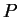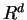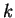Next: What are the faces Up: Convex Polyhedron Previous: Convex Polyhedron   Contents

## What is convex polytope/polyhedron?

A subsetofis called a convex polyhedron if it is the set of solutions to a finite system of linear inequalities, and called convex polytope if it is a convex polyhedron and bounded. When a convex polyhedron (or polytope) has dimension, it is called a-polyhedron (-polytope). For the sequel, we might omit convex for convex polytopes and polyhedra, and call them simply polytopes and polyhedra.

Komei Fukuda 2004-08-26